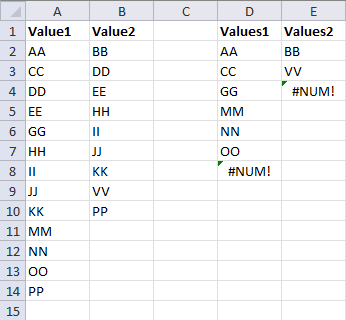Author: Oscar Cronquist Article last updated on November 26, 2013

There are text values in column A and column B. The question is how do you compare the values in these two columns? More specifically, filtering values existing only in column A (or column B)?The array formula in cell D2 extracts values existing only in column A, compared to column B:

=INDEX(\$A\$2:\$A\$14, SMALL(IF(COUNTIF(\$B\$2:\$B\$10, \$A\$2:\$A\$14)=0, MATCH(ROW(\$A\$2:\$A\$14), ROW(\$A\$2:\$A\$14)), ""),ROW(A1)))

The array formula in cell E2 extracts values existing only in column B, compared to column A:

=INDEX(\$B\$2:\$B\$10, SMALL(IF(COUNTIF(\$A\$2:\$A\$14, \$B\$2:\$B\$10)=0, MATCH(ROW(\$B\$2:\$B\$10), ROW(\$B\$2:\$B\$10)), ""),ROW(A1)))

How to enter an array formula

1. Copy array formula (Ctrl + c)
2. Select cell D2
3. Click in formula bar
4. Paste array formula (Ctrl + v)
5. Press and hold CTRL + Shift
6. Press Enter

The formula is now an array formula. See the curly brackets, they tell you it is an array formula. Don´t enter the curly brackets yourself, they appear if you enter it correctly, like this:

{=INDEX(\$A\$2:\$A\$14, SMALL(IF(COUNTIF(\$B\$2:\$B\$10, \$A\$2:\$A\$14)=0, MATCH(ROW(\$A\$2:\$A\$14), ROW(\$A\$2:\$A\$14)), ""), ROW(A1)))}

How to copy array formula

1. Select cell D2
2. Copy (Ctrl + c)
3. Select cell range D3:D8
4. Paste (Ctrl + v)

### Explaining array formula in cell D2

Step 1 - Count the number of values in cell range A2:A14 using the values in cell range B2:B10

COUNTIF(\$B\$2:\$B\$10,\$A\$2:\$A\$14)

Step 2 - Check if they are equal to 0 (zero)

COUNTIF(\$B\$2:\$B\$10, \$A\$2:\$A\$14)=0

Step 3 - If they are equal to zero, return the corresponding relative row number

IF(COUNTIF(\$B\$2:\$B\$10, \$A\$2:\$A\$14)=0, MATCH(ROW(\$A\$2:\$A\$14), ROW(\$A\$2:\$A\$14)), "")

Step 4 - Return the k-th smallest row number

SMALL(IF(COUNTIF(\$B\$2:\$B\$10, \$A\$2:\$A\$14)=0, MATCH(ROW(\$A\$2:\$A\$14), ROW(\$A\$2:\$A\$14)), ""), ROW(A1))

Step 5 - Return value

=INDEX(\$A\$2:\$A\$14, SMALL(IF(COUNTIF(\$B\$2:\$B\$10, \$A\$2:\$A\$14)=0, MATCH(ROW(\$A\$2:\$A\$14), ROW(\$A\$2:\$A\$14)), ""), ROW(A1)))

### Interesting articles

Filter values existing in range 1 but not in range 2 using array formula

Filter text values existing in range 1 but not in range 2 using array formula

I have also written an article about comparing records between two data tables:
Compare two lists of data: Filter records existing in only one list

Filter values existing in column 1 but not in column 2.xlsx

IF(logical_test, [value_if_true], [value_if_false])
Checks whether a condition is met, and returns one value if TRUE, and another value if FALSE

INDEX(array,row_num,[column_num])
Returns a value or reference of the cell at the intersection of a particular row and column, in a given range

SMALL(array,k)
Returns the k-th smallest number in this data set.

ROW(reference)
Returns the rownumber of a reference

MATCH(lookup_value, lookup_array, [match_type]
Returns the relative position of an item in an array that matches a specified value

COUNTIF(range,criteria)
Counts the number of cells within a range that meet the given condition